# MP Board Class 10th Maths Solutions Chapter 4 Quadratic Equations Ex 4.4

In this article, we will share MP Board Class 10th Maths Book Solutions Chapter 4 Quadratic Equations Ex 4.4 Pdf, These solutions are solved subject experts from the latest edition books.

## MP Board Class 10th Maths Solutions Chapter 4 Quadratic Equations Ex 4.4Question 1.
Find the nature of the roots of the following quadratic equations. If the real roots exist, find them:
(i) 2x2 – 3x + 5 = 0
(ii) 3x2 – 4$$\sqrt{3} x$$ + 4 = 0
(iii) 2x2 – 6x + 3 = 0
Solution:
(i) Comparing the given quadratic equation with ax2 + bx + c = 0, we get a = 2, b = -3, c = 5
b2 – 4 ac = (-3)2 – 4(2)(5) = 9 – 40 = -31 < 0
Since b2 – 4ac is negative.
∴ The given quadratic equation has no real roots.

(ii) Comparing the given quadratic equation with ax2 + bx + c = 0, we get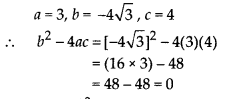Since, b2 – 4ac is zero.
∴ The given quadratic equation has two real roots which are equal. Hence, the roots are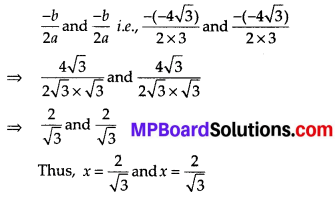(iii) Comparing the given quadratic equation with ax2 + bx + c = 0, we get a = 2,b = -6,c = 3
∴ b2 – 4ac = (-6)2 – 4(2)(3)
= 36 – 24 = 12 > 0
Since, b2 – 4ac is positive.
∴ The given quadratic equation has two real and distinct roots, which are given by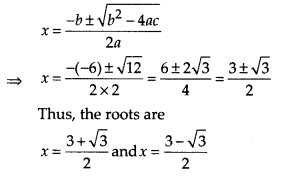Question 2.
Find the values of k for each of the following quadratic equations, so that they have two equal roots.
(i) 2x2 + kx + 3 = 0
(ii) kx(k – 2) + 6 = 0
Solution:
(i) 2x2 + kx + 3 = 0
Here, a = 2, b = k, c = 3
b2 – 4ac = (k)2 – 4(2) (3)
= k2 – 24
∵ For a quadratic equation to have equal root, b2 – 4ac = 0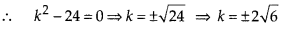(ii) kx(k – 2) + 6 = 0
Comparing kx2 – 2kx + 6 = 0 with ax2 + bx + c, we get
a = k, b = -2k, c = 6
∴  b2 – 4ac = (-2k)2 – 4(k)(6) = 4k2 – 24k
Since, the roots are equal
∴  b2 – 4ac ⇒ 4k2 – 24k = 0.
⇒ 4k(k – 6) = 0
⇒ 4k = 0 or k – 6 = 0
⇒ k = 0 or k = 6
But k cannot be 0, otherwise, the given equation is not quadratic. Thus, the required value of k is 6.Question 3.
Is it possible to design a rectangular mango grove whose length is twice its breadth, and the area is 800 m2 ? If so, find its length and breadth.
Solution:
Let the breadth be x m.
∴ Length = 2x m
Now, Area = Length × Breadth = 2x × x m2 = 2x2 m2
According to the given condition,
2x2 = 800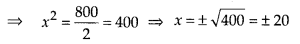Therefore, x = 20 or x = -20
But x = -20 is not possible
[ ∵ Breadth cannot be negative]
∴ x = 20 ⇒ 2x = 2 × 20 = 40
Thus, length = 40 m and breadth = 20 mQuestion 4.
Is the following situation possible? If so, determine their present ages. The sum of the ages of two friends is 20 years. Four years ago, the product of their ages in years was 48.
Solution:
Let the age of one of the friends be ‘x’.
Age of another friend is (20 – x).
4 years back age of 1st friend is (x – 4)
4 years back age of 2nd friend = (20 – x – 4) = (16 – x)
Product of their ages is 48.
∴ (x – 4) (16 – x) = 48
16x – x2 – 64 + 4x = 48
-x2 + 20x – 64 = 48
-x2 + 20x – 64 – 48 = 0
-x2 + 20x – 112 = 0
x2 – 20x + 112 = 0
Here, a = 1, b = -20, c = 112
b2 – 4ac = (-20)2 – 4( 1)( 112) = 400 – 448 = – 48
Here, b2 – 4ac = -48 < 0.
∴ It has no real roots.
∴ This situation is not possible.Question 5.
Is it possible to design a rectangular park of perimeter 80 m and area 400 m2? If so, find its length and breadth.
Solution:
Let the breadth of the rectangle be x m.
Since, the perimeter of the rectangle = 80 m
∴ 2(Length + breadth) = 80
2(Length + x) = 80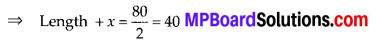⇒ Length = (40 – x) m
∴ Area = (40 – x) × x m2 = (40x – x2) m2
According to the given condition,
Area of the rectangle = 400 m2
⇒ 40x – x2 = 400
⇒ – x2 + 40x – 400 = 0
⇒ x2 – 40x +400 = 0 …(1)
Comparing equation (1) with ax2 + bx + c = 0, we get
a = 1, b = -40, c = 400